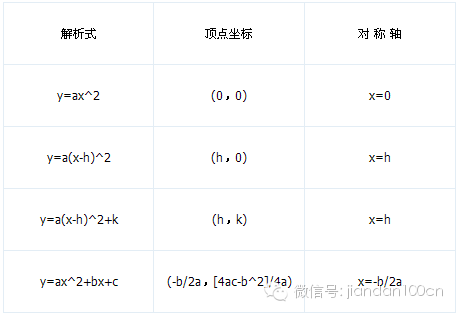### 问吧

\',msg=md5(3.14),email=\'...[详细]

### 初中数学二次函数知识点总结

I.定义与定义表达式

abc为常数，a0，且a决定函数的开口方向，a>0时，开口方向向上，a<0时，开口方向向下,IaI还可以决定开口大小,IaI越大开口就越小,IaI越小开口就越大.）则称yx的二次函数。

II.二次函数的三种表达式

h=-b/2a   k=(4ac-b^2)/4a   x,x=(-b±√b^2-4ac)/2a

III.二次函数的图像

IV.抛物线的性质

1.抛物线是轴对称图形。对称轴为直线    x = -b/2a

2.抛物线有一个顶点P，坐标为：P ( -b/2a (4ac-b^2)/4a )-b/2a=0时，Py轴上；当Δ= b^2-4ac=0时，Px轴上。

3.二次项系数a决定抛物线的开口方向和大小。

a0时，抛物线向上开口；当a0时，抛物线向下开口。|a|越大，则抛物线的开口越小。

4.一次项系数b和二次项系数a共同决定对称轴的位置。

ab同号时（即ab0），对称轴在y轴左；

ab异号时（即ab0），对称轴在y轴右。

5.常数项c决定抛物线与y轴交点。

6.抛物线与x轴交点个数

Δ= b^2-4ac0时，抛物线与x轴有2个交点。

Δ= b^2-4ac=0时，抛物线与x轴有1个交点。

Δ= b^2-4ac0时，抛物线与x轴没有交点。X的取值是虚数（x= -b±√b^24ac 的值的相反数，乘上虚数i，整个式子除以2a

V.二次函数与一元二次方程

y=0时，二次函数为关于x的一元二次方程（以下称方程），即ax^2+bx+c=0

1．二次函数y=ax^2y=a(x-h)^2y=a(x-h)^2 +ky=ax^2+bx+c(各式中，a0)的图象形状相同，只是位置不同，它们的顶点坐标及对称轴如下表：h>0时，y=a(x-h)^2的图象可由抛物线y=ax^2向右平行移动h个单位得到，

h<0时，则向左平行移动|h|个单位得到．

h>0,k>0时，将抛物线y=ax^2向右平行移动h个单位，再向上移动k个单位，就可以得到y=a(x-h)^2 +k的图象；

h>0,k<0时，将抛物线y=ax^2向右平行移动h个单位，再向下移动|k|个单位可得到y=a(x-h)^2+k的图象；

h<0,k>0时，将抛物线向左平行移动|h|个单位，再向上移动k个单位可得到y=a(x-h)^2+k的图象；

h<0,k<0时，将抛物线向左平行移动|h|个单位，再向下移动|k|个单位可得到y=a(x-h)^2+k的图象；

2．抛物线y=ax^2+bx+c(a0)的图象：当a>0时，开口向上，当a<0时开口向下，对称轴是直线x=-b/2a，顶点坐标是(-b/2a[4ac-b^2]/4a)

3．抛物线y=ax^2+bx+c(a0)，若a>0，当≤ -b/2a时，yx的增大而减小；当≥ -b/2a时，yx的增大而增大．若a<0，当≤ -b/2a时，yx的增大而增大；当≥ -b/2a时，yx的增大而减小．

4．抛物线y=ax^2+bx+c的图象与坐标轴的交点：

(1)图象与y轴一定相交，交点坐标为(0c)

(2)当△=b^2-4ac>0，图象与x轴交于两点A(x₁，0)B(x₂，0)，其中的x1,x2是一元二次方程ax^2+bx+c=0

(a0)的两根．这两点间的距离AB=|x-x|

5．抛物线y=ax^2+bx+c的最值：如果a>0(a<0)，则当x= -b/2a时，y最小()=(4ac-b^2)/4a

6．用待定系数法求二次函数的解析式

(1)当题给条件为已知图象经过三个已知点或已知xy的三对对应值时，可设解析式为一般形式：

y=ax^2+bx+c(a0)

(2)当题给条件为已知图象的顶点坐标或对称轴时，可设解析式为顶点式：y=a(x-h)^2+k(a0)

(3)当题给条件为已知图象与x轴的两个交点坐标时，可设解析式为两根式：y=a(x-x)(x-x)(a0)

7．二次函数知识很容易与其它知识综合应用，而形成较为复杂的综合题目。因此，以二次函数知识为主的综合性题目是中考的热点考题，往往以大题形式出现．

### 更多相关内容### 扬格外语承诺

“想同学之所想，急家长之所急”是扬格教育服务的理念。学校采用四位一体的服务模式，即：教务主管+课程顾问+班主任+任课教师的综合服务模式。

“选择扬格，梦想飞扬!”是所有扬格人的承诺。中心课程包教包会，教学质量有保障。开课前无条件转班、调课。学校采用学员否决制，由学员对教学团队进行严格考核!